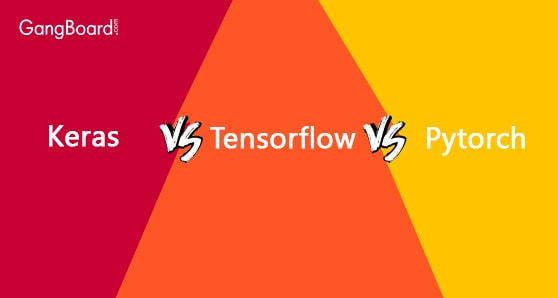# Keras Vs Tensorflow Vs Pytorch

May 14th, 2019

## Keras Vs Tensorflow Vs Pytorch

Keras is a library framework based developed in Python language. This library is an open-source neural-network library framework. This library is applicable for the experimentation of deep neural networks. Tensorflow is an open-source software library for differential and dataflow programming needed for different various kinds of tasks. This library is applicable for developing neural networks. Pytorch is based on the Torch library. This library is an open source machine learning library for programming written in python coding language.

### Why Keras?

This is another library framework which is written in Python code language. Keras library framework is fully capable of running on Microsoft Cognitive Toolkit, Theano, PlaidML, and Tensorflow. While developing this framework, the emphasis was given on it being modular, extensive, and user-friendly.

The framework would consist of several neural network building blocks. These building blocks would include objectives, optimizers, activation functions, and layers. This library would also contain several tools that would help while working with text data and images. Along with the general neural network, Keras would also support recurrent neural and convolutional networks. This library also supports common utility layers.

### Why Tensorflow?

Tensorflow is a library framework that is applicable for machine learning applications. This framework is a mathematical library used for numerical computation by applying data from the flow graph. The edges of the graph would represent multidimensional data arrays and nodes would represent various mathematical representations. It would teach neural networks about the mathematical symbol, image recognition, and partial differentiation. This library is fully capable of running on multiple GPUs and CPUs.

The architecture of the framework is very flexible in nature. This framework could be implemented either on desktops, servers’ clusters or edge and mobile devices. This library framework was initially made for the internal use of Google. This framework might also support Haskell, Julia, Rust, C#, Scala, Crystal, and OCami. Tensorflow is also used as the foundation of the automated image capturing software.

### Why Pytorch?

This framework is a machine learning library which is applicable for application such as natural language processing. Pytorch is also applicable for building various types of applications. This library framework has two important features. The first feature of the library would be the automatic differentiation for training and building of the deep neural network. The network would base upon the auto grad system.

The second feature would be the tensor computational ability with support from high power GPU acceleration. Pytorch has three modules of operations. They are the Optimum Module, Autograd Module, and nn Module. Each module has its own specific functions and applications. For example, Optimum Module is for implementing various types of an algorithm for the development of the neural network. And the nn Module is for defining all complex low-level neural networks.

### Difference Between Keras, Tensorflow and Pytorch

#### Pytorch

Keras framework is an open source neural network library.

Tensorflow is a free software library. This library is open source in nature.

The Pytorch framework is an open source machine learning library.

Keras library is also for Python based coding.

Tensorflow library is compatible with various coding languages such as C, C++, Java, etc.

The Pytorch library is only for the Python-based coding.

This framework is for performing fast experiments with neural networks. This framework is for teaching the machine about various computational methods. This framework is for building a neural network and for natural language processing.
Keras library has very high-level API which could run on CNTK and Theano.. Tensorflow library has both low-level and high-level APIs. The Pytorch library has only low-level APIs that would focus on the working of array expression.
Keras is popular due to the syntactic simplicity and user-friendly nature. Tensorflow is famous for its fast computational ability across several platforms. Pytorch is generally famous for academic research purposes. It would also assist in deep learning applications.
The speed of Keras slower while comparing with Tensorflow and Pytorch. The speed of Tensorflow is very fast and would provide high performance. The speed and performance of Pytorch are very much similar to the Tensorflow.
The architecture of the Keras is pretty simple. The architecture of the Tensorflow is a little complex and would be a little difficult to understand. The architecture of the Pytorch is pretty complex and it would be very difficult for any beginners.

In Keras, the developer would not require to debug any simple network.

The process of debugging in Tensorflow is very difficult. Debugging abilities of Pytorch is much better when compared to Keras and Tensorflow.
Keras uses small datasets for processing as the processing speed of the library is very slow. Tensorflow is fully capable of handling large datasets, as the processing speed of the library is very fast. Pytorch is also able to handle large datasets and high-performance tasks.
All codes of the Keras framework are single line code. The size of the codes of Tensorflow is small in size to increase its accuracy. All codes for Pytorch consist of several lines.
Social Share: